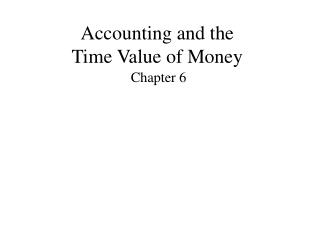DownloadDownload PresentationAccounting and the Time Value of Money

# Accounting and the Time Value of Money

Télécharger la présentation## Accounting and the Time Value of Money

- - - - - - - - - - - - - - - - - - - - - - - - - - - E N D - - - - - - - - - - - - - - - - - - - - - - - - - - -
##### Presentation Transcript

1. Basic Time Value Concept • The time value of money is the relationship between time and money. • According to the present value of money concept, a dollar today is worth more than a dollar in the future. • This concept is used extensively to choose among alternative investment proposals.

2. Accounting Applications • Notes • Leases • Pensions • Long-term assets • Sinking funds • Business combinations • Disclosures • Installment contracts

3. Fundamental Variables • Interest rate: A percentage rate usually expressed as an annual rate of return. • Time: the number of years or fractional portion of a year (periods) that amounts compound. • Present Value: The value now (present) of a set of future cash flows. • Future Value: The value at a given future date of cash invested (may be multiple FV’s).

4. Basic Time Diagram

5. Interest Rates • Specified in contracts • Stated • Coupon • Nominal • Face • Demanded by investors • Effective • Discount • Required rate of return

6. Determining the effective rate The appropriate interest rate depends on: • the pure rate of interest • expected inflation rate of interest • credit risk rate of interest The higher the credit risk, the higher the interest rate.

7. Simple vs. Compound interest • Simple interest is determined using only the original principal amount. principal x interest rate (%) x time • Compound interest is determined using: • the principal, and any interest accrued (earned and not withdrawn or paid). • Compound interest is used in virtually all time value applications.

8. The basic calculations • Future value of \$1 • Present value of \$1 • Future value of an ordinary annuity of \$1 • Present value of an ordinary annuity of \$1 • Future value of an annuity due of \$1 • Present value of an annuity due of \$1

9. Single cash flow problems Typically one of two types: • Computing a future value of a known single sum present value. • Computing a present value of a known single sum future value. • FV = PV*(1+i)^n or PV = FV/(1+i)^n i = interest rate per period n = the number of periods

10. Single cash flow FV example Given: • Amount of deposit today (PV): \$50,000 • Interest rate 8% • Frequency of compounding: Quarterly • Time outstanding: 5 years What is the future value of this single sum?

11. Single cash flow PV example Given: • Amount of deposit end of 3 years: \$100,000 • Interest rate (discount) rate: 12% • Frequency of compounding: Quarterly • Time outstanding: 3 years What is the present value of this single sum?

12. Annuity Calculations An annuity requires that: • the periodic payments or receipts (rents) always be of the same amount, • the interval between such payments or receipts be the same, and • the interest be compounded once each interval.

13. Types of annuities Annuities may be broadly classified as: • Ordinary annuities: where the rents occur at the end of the period. • Annuities due: where rents occur at the beginning of the period.

14. Future Value of an Ordinary Annuity Given: • Deposit made at the end of each period: \$5,000 • Compounding: Annual • Number of periods: Five • Interest rate: 12% • What is future value of these deposits?

15. Present Value of an Ordinary Annuity Given: • Rental receipts at the end of each period: \$6,000 • Compounding: Annual • Number of periods (years): 5 • Interest rate: 12% • What is the present value of these receipts?

16. Complex Situations Deferred Annuities: • Rents begin after a specified number of periods. Valuation of Long-term Bonds: • Two cash flows: principal paid at maturity and periodic interest payments

17. Expected Cash Flows • Introduced by SFAC No. 7 • Uses a range of cash flows. • Incorporates the probabilities of those cash flows to arrive at a more relevant measurement of present value.